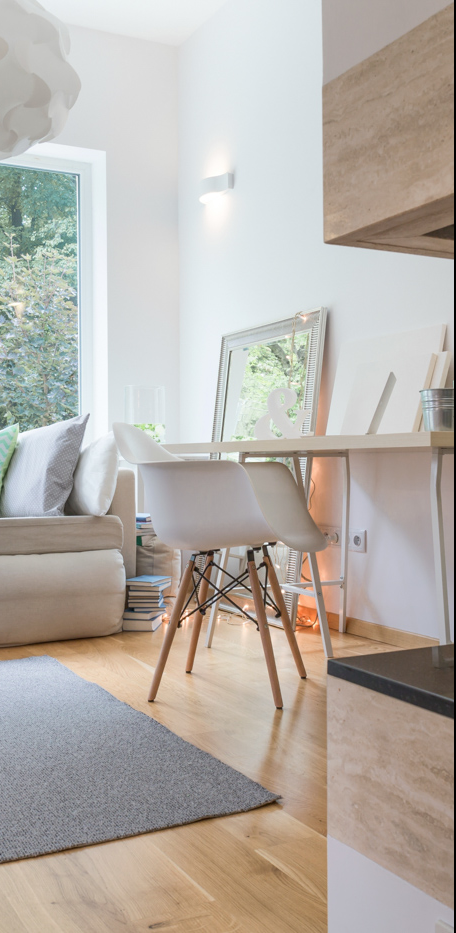Your monthly payment (PITI) is the sum of four items - the principal on the loan (P), the interest on the loan (I), property taxes {T), and homeowner' s insurance (I). In many cases when you are putting less than 20% down, you will also have to pay Private Mortgage Insurance, or PMI. To predict your monthly payment for a 30-year fixed rate loan, use the table below to determine the principal and interest part of the payment.

Simply divide the loan amount by 1,000 and then multiply that figure by the appropriate interest rate factor from the table. To that sum add 1112th of the amount of your yearly taxes and 1112th the amount of your yearly insurance. To calculate your monthly PMI, you multiply .52% by the loan amount, and then divide by 12.

### Example

You have found a home that you like that listed at 209,000 and you have reached a negotiated contract with the seller to buy the home for a sales price of \$200,000. If you are putting 5%

down, or \$10,000, then your loan amount is \$190,000. Assuming a conventional loan and an interest rate of 7%, your monthly "PI" on a \$190,000 loan would be:

\$190.000 = 190

1,000

190 X 6.65 = 1,263.50

This does not end your calculation. To your PI, you need to add your taxes, your hazard insurance, and your PMI, if applicable. Using estimated annual taxes of \$4,000 (Area lB), annual hazard insurance of \$900, and annual PMI of \$988, your total PITH is:

1,263.50 (PI)

+ 333.34 (l/12 of taxes)

+ 75.00 (l/12 ofhazard)

+ 82.34 (l/12 of PMI}

= 1754.18

PRINCIPAL AND INTEREST PAYMENT TABLE
If your interest rate is: PI Factor is:
6.00%6.00
6.50%6.32
7.00%6.65
7.50%6.99
8.00%7.34
8.50%7.69
9.00%8.05
9.50%8.41
10.0%8.78
10.5%9.15
11.0%9.52